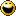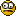# SurvivingInfidelity.com® > Off Topic

You are not logged in. Login here or register.

### Any Math Folks Still Up?

StillLivin posted 10/4/2018 22:58 PM

I sick at word problems and functions. Combine the two and forget it. Anyone want to point me in the right direction?
The problem is: An accident at an oil drilling platform is causing a circular-shaped oil slick to form. The volume of the oil slick is roughly given by V(r)= 0.08 x pi x r(squared), where r is the radius of the slick in feet. In turn, the radius is increasing over time according to the function r(t) = 0.5t, where t is measured in minutes. Find (V of r)(t), and give a practical interpretation of what this function tells you. After how many minutes will the volume of the slick be 225 cubic feet?

Any takers? All I know is the volume formula. 🤷‍♀️

still-living posted 10/5/2018 06:11 AM

I'm not a math major but I will attempt to help.

Assume 0.08 is in feet.
Pi is 3.14 with no units

2((225 / (0.08 x 3.14))^(1/2)) = 60 minutes.

0.08 x 3.14 = 0.251
225 / 0.251 = 896
square root of 896 = 29.9
2 x 29.9 = 60 minutes

Leak rate was 225 cubit feet in the first hour.

or, 7.48 us liquid gallons equals 1 cubic foot
Leak rate was 1,680 gallons in the first hour.

or \$50 per barrel, and 42 gallons per barrel.
Leak rate is \$2000 per hour! Plus cleanup!

Leak rate is growing exponentially over time. In other words, the leak rate will be \$4000 more in the next hour! \$16k in the next hour.

Fix your problems while they are small, before they turn into bigger problems!

[This message edited by still-living at 6:37 AM, October 5th (Friday)]

barcher144 posted 10/5/2018 12:32 PM

I'm an engineer...

The volume formula is:

V = 0.08 * pi * r * r

r = 0.5 * t

So, plug the second equation into the first equation:

V = 0.08 * pi * (0.05 * t) * (0.05 * t)

Simplifying:

V = 0.0002 * pi * t * t

(practical interpretation: the volume increases as a function of time-squared, meaning that it accelerates over time... it's not linear).

Now, if V = 225, then you can solve for t:

225 = 0.0002 * pi * t * t

358098.9 = t * t

t = 598.4 minutes

ZenMumWalking posted 10/5/2018 12:56 PM

barcher put in .05 where it should have been .5, so numerically you have:

V = 0.08 * pi * (0.5 * t) * (0.5 * t)
=> V = 0.02 * pi * t * t
=> Now, if V = 225, then you can solve for t:

225 = 0.02 * pi * t * t

and approximating pi by 3.14, you get

t * t = 225/(0.02 * 3.14) = 3582.8
=> t = sqrt(3582.8) = approx 59.86 (up to round-off error)

In general, the volume formula for a cylinder is pi*(r squared)*(height of the cylinder), so here the height is 0.08, which we are assuming is constant across time.

PlanC posted 10/5/2018 14:52 PM

Im not an engineer, but I like humorous science fiction, and accordingly was going to suggest that the answer is 42.

ZenMumWalking posted 10/5/2018 15:52 PM

Im not an engineer

Nor do you play one on tv I assume.

but I like humorous science fiction, and accordingly was going to suggest that the answer is 42.Zigin posted 10/5/2018 20:54 PM

Translation: There are some seriously smart men and women on this board.

barcher144 posted 10/5/2018 21:05 PM

I'm an engineer...

Translation: I don't read very carefully.

Emotionalhell posted 10/5/2018 21:10 PM

BearlyBreathing posted 10/5/2018 21:42 PM

42 is the answer... to everything

Greeneyesbluezy posted 10/5/2018 22:37 PM

Can I say I hate you guys? Like totally hate you??!

Jokes aside, best of luck!!!

StillLivin posted 10/6/2018 10:19 AM

Thank you everyone. Sorry I didn't come back to respond sooner. I did exactly what you said Barcher, but I was doubting myself. I got a 100 on the homework so I guess I'm not quite as dumb as I thought. Yikes I'm still in shock I got all the answers correct because i seriously don't know for certain when I've guessed right on the formulas.
You guys are hilarious!!!

still-living posted 10/6/2018 10:25 AM

But what about significant digits?WhatsRight posted 10/11/2018 18:56 PM

ZenMumWalking...BAM!!!